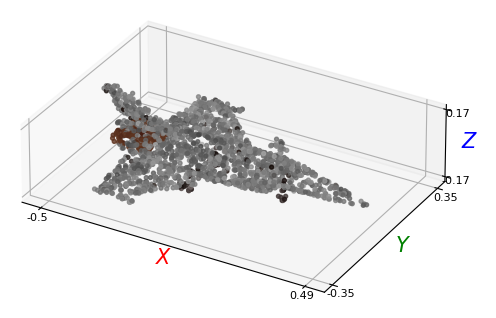We show here how to use the basic HDF5 pointcloud dataloader that we provide.

```from demo_utils_3D import *
import utils3D.plot as plt_utils

ROOT_DIR = "./3D_PC_data/"
```

## Working with stylized pointclouds#

To load pre-sampled pointclouds, we provide the `StylizedShapeLoader` class. The n_points parameter enables sampling of pointclouds when loading the shapes.

It can be instantiated with the load_mesh parameter toggled to interactively visualize the shapes with a placeholder style.

Meshes fetched by the loader are trimesh.Trimesh instances that contain all relevant geometry information.

```"""

Args:
----
root_dir:          Root directory containing HDF5 files.
split:             One of {train, valid}.
semantic_level:    Semantic level to use for segmentations. One of {fine, medium, coarse}
num_points:        Number of points to sample.
transform:         Data transformations.
half_precision:    Use half precision floats.
normalize_points:  Normalize point clouds.
is_rgb:            The HDF5 to load has RGB features.
"""

split="valid",
semantic_level="fine",
num_points=2048,
transform=None,
half_precision=False,
normalize_points=False,
is_rgb=True)

# Iterating over the dataset
for k in range(len(valid_dataset)):
shape_id, style_id, shape_label, points, points_part_labels, points_mat_labels = valid_dataset[k]
# Separate points and colors
points_xyz = points[:, :3]
points_col = points[:, 3:]

# Print unique part labels
print("Unique part labels: ", np.unique(points_part_labels))

# Print unique material labels
print("Unique material labels: ", np.unique(points_mat_labels))
print()

# Print shape ID
print("Shape ID: ", shape_id)
print("Style ID: ", style_id)
print("Shape label: ", shape_label)

break
```
```Unique part labels:  [  1   3   8   9  10  11 271 273]
Unique material labels:  [2 6 8]

Shape ID:  00_004
Style ID:  0
Shape label:  0
```
```print("Visualizing part labels:")
plt_utils.plot_pointclouds([np.array(points_xyz)],
point_labels=[np.array(points_part_labels)],
size=15,
cmap='viridis',
point_size=8)
```
```Visualizing part labels:
```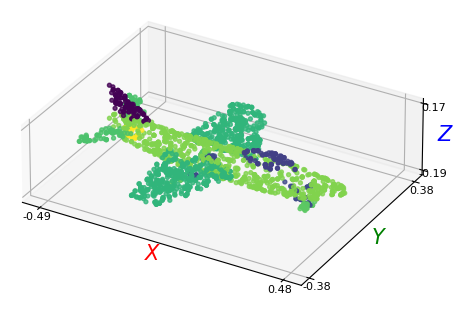```print("Visualizing material labels:")
plt_utils.plot_pointclouds([np.array(points_xyz)],
point_labels=[np.array(points_mat_labels)],
size=15,
cmap='viridis',
point_size=8)
```
```Visualizing material labels:
```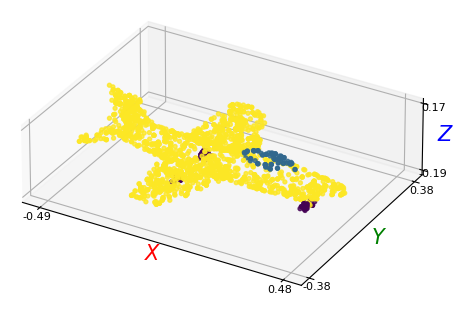```print("Visualizing RGB pointclouds:")
plt_utils.plot_pointclouds([np.array(points_xyz)],
colors=[np.array(points_col)],
size=15,
cmap='viridis',
point_size=8)
```
```Visualizing RGB pointclouds:
```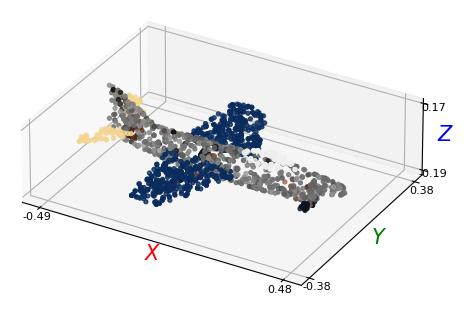Hint

In this first release, we only provide pre-extracted pointclouds for the first `10` compositions of our dataset. There is thus no `n_compositions` option modulating for the number of loaded styles per model. If you want to explore more compositions, please refer to our documentation on sampling your own dataset.

## Fetching a stylized shape#

We enable directly fetching a stylized shape’s pointcloud from the dataloader by using its `shape_id` and `style_id`, to enable better compatibility with the `2D` streamed loader.

We illustrate this in the example below:

```# Recovering the stylized airplane above
_, _, _, points, _, _ = valid_dataset.get_stylized_shape("00_004", "0")
points_xyz = points[:, :3]
points_col = points[:, 3:]
plt_utils.plot_pointclouds([np.array(points_xyz)],
colors=[np.array(points_col)],
size=15,
cmap='viridis',
point_size=8)
```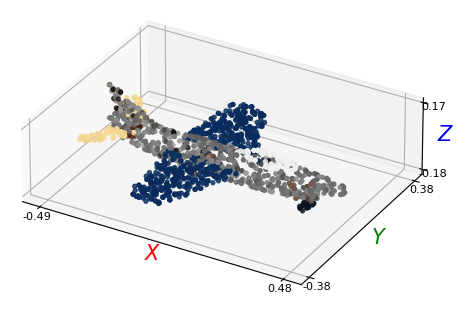Hint

The section below is not relevant, as we have now released the test set labels, as our CVPR 2023 challenge is over. You can access the test data loader just as any other split, using the `split="test"` parameter. For more information, please take a look at the documentation on the 2D dataloaders.

The test HDF5 files that we provide are stripped of all ground truth data. They only contain:

• `a)` the sampled pointcloud

• `b)` the stylized shape identifiers (`shape_id`, `style_id`)

To iterate over the test dataloader, you need a special dataloader, `EvalLoader_PC`, which only fetches these fields form the data shards.

We illustrate below its usage:

```"""

Args:
----
"""

semantic_level="coarse",
num_points=2048)

# Iterating over the dataset
for k in range(len(test_dataset)):
shape_id, style_id, points = test_dataset[k]
# Separate points and colors
points_xyz = points[:, :3]
points_col = points[:, 3:]

# Print shape ID
print("Shape ID: ", shape_id)
print("Style ID: ", style_id)
break

plt_utils.plot_pointclouds([np.array(points_xyz)],
colors=[np.array(points_col)],
size=15,
cmap='viridis',
point_size=8)
```
```Shape ID:  00_000
Style ID:  0
```